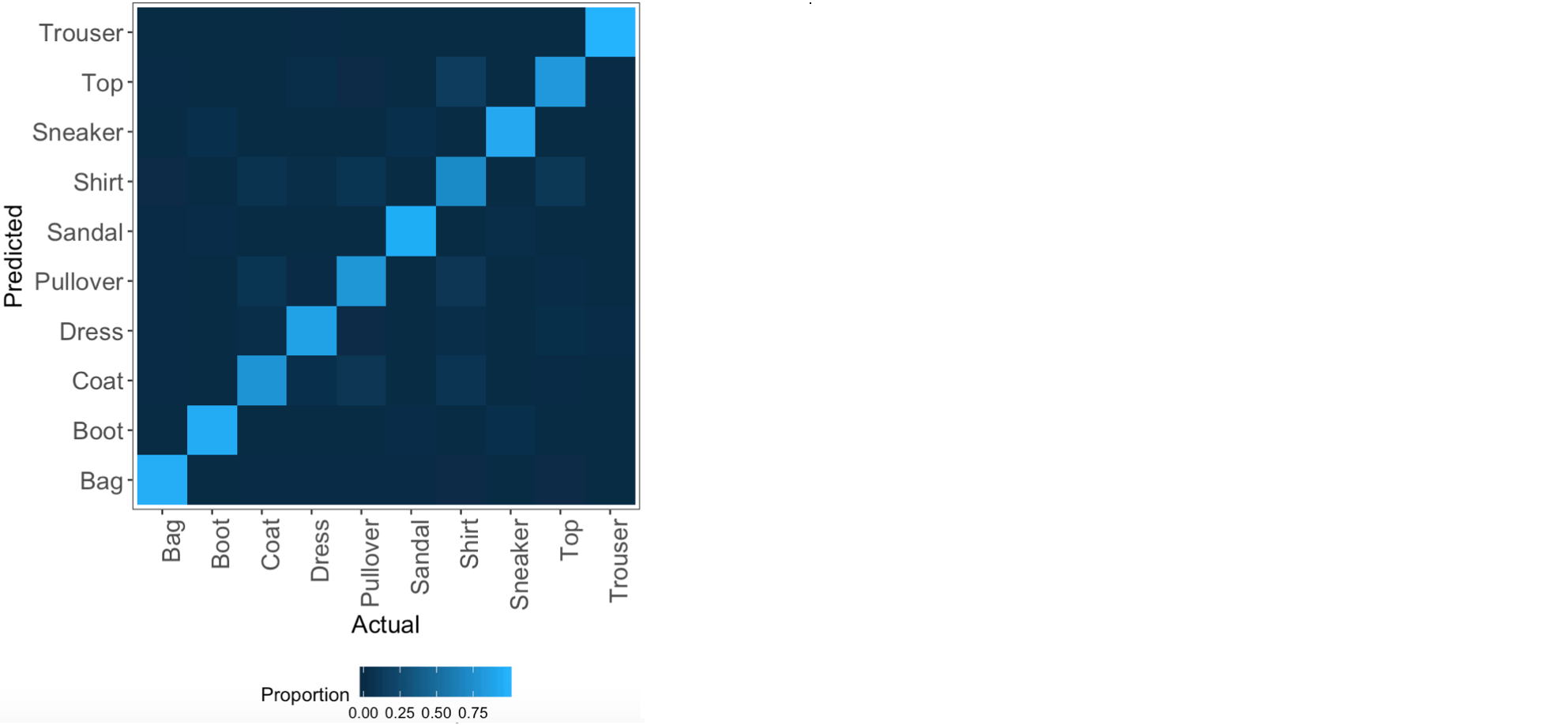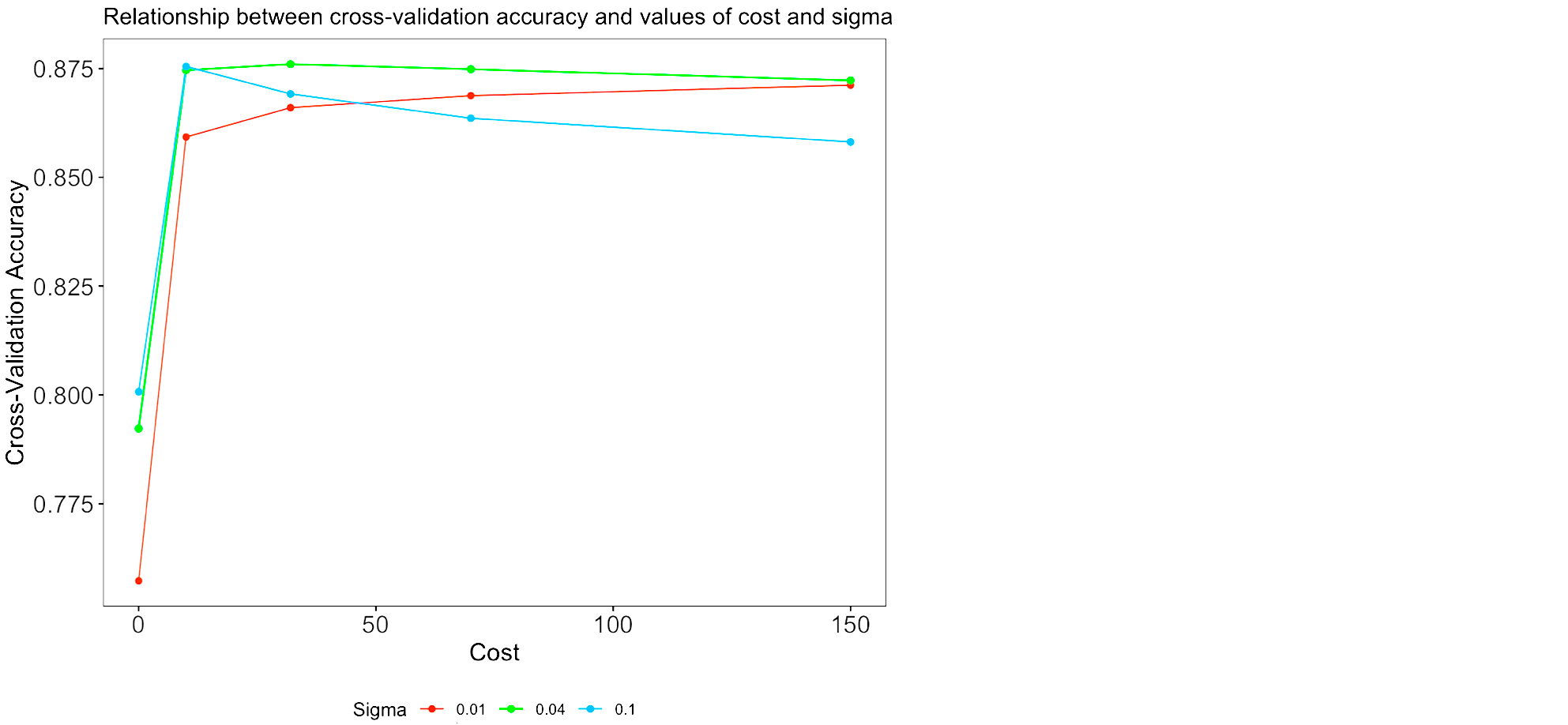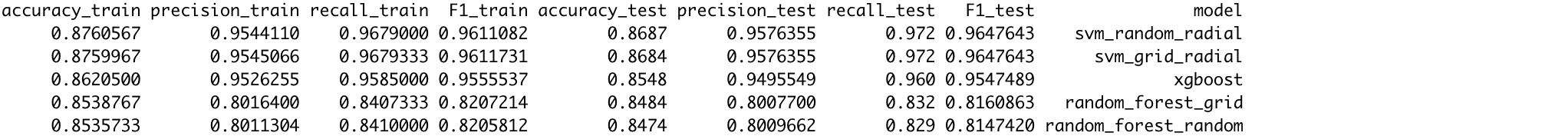Want to share your content on R-bloggers? click here if you have a blog, or here if you don't.

Florianne Verkroost is a Ph.D. candidate at Nuffield College at the University of Oxford. She has a passion for data science and a background in mathematics and econometrics. She applies her interdisciplinary knowledge to computationally address societal problems of inequality.

This is the fourth and final post in a series devoted to comparing different machine learning methods for predicting clothing categories from images using the Fashion MNIST data by Zalando. In the first post, we prepared the data for analysis and built a Python deep learning neural network model to predict the clothing categories of the Fashion MNIST data. In Part 2, we used principal components analysis (PCA) to compress the clothing image data down from 784 to just 17 pixels. In Part 3 we saw that gradient-boosted trees and random forests achieve relatively high accuracy on dimensionality-reduced data, although not as high as the neural network. In this post, we will fit a support vector machine, compare the findings from all models we have built and discuss the results. The R code for this post can be found on my Github repository.

# Support Vector Machine

Support vector machines (SVMs) provide another method for classifying the clothing categories in the Fashion MNIST data. To better understand what SVMs entail, we’ll have to go through some more complex explanations –mainly summarizing James et. al. (2013)– so please bear with me! The figure below might help you in understanding the different classifiers I will discuss in the next sections (figures taken from here, here and here).For an $$n \times p$$ data matrix and binary outcome variable $$y_i \in \{-1, 1\}$$, a hyperplane is a flat affine subspace of dimension $$p – 1$$ that divides the $$p$$-dimensional space into two halves, defined by $$\beta_0 + \beta_1 X_1 + \dots + \beta_p X_p$$. An observation in the test data is assigned an outcome class depending on which side of the perfectly separating hyperplane it lies, assuming that such a hyperplane exists. Cutoff $$t$$ for an observation’s score $$\hat{f}(X) = \hat{\beta}_1 X_1 + \hat{\beta}_2 X_2 + \dots + \hat{\beta}_p X_p$$ determines which class it will be assigned to. The further an observation is located from the hyperplane at zero, the more confident the classifier is about the class assignment. If existent, an infinite number of separating hyperplanes can be constructed. A good option in this case would be to use the maximal margin classifier (MMC), which maximizes the margin around the midline of the widest strip that can be inserted between the two outcome classes.

If a perfectly separating hyperplane does not exist, “almost separating” hyperplanes can be used by means of the support vector classifier (SVC). The SVC extends the MMC as it does not require classes to be separable by a linear boundary by including slack variables $$\epsilon_i$$ that allow some observations to be on the incorrect side of the margin or hyperplane. The extent to which incorrect placements are done is determined by tuning parameter cost $$C \geq \sum_{i=1}^{n} \epsilon_i$$, which thereby controls the bias-variance trade-off. The SVC is preferable over the MMC as it is more confident in class assignments due to the larger margins and ensures greater robustness as merely observations on the margin or violating the margin affect the hyperplane (James et al., 2013).

Both MMCs and SVCs assume a linear boundary between the two classes of the outcome variable. Non-linearity can be addressed by enlarging the feature space using functions of predictors. Support vector machines combine SVCs with non-linear (e.g. radial, polynomial or sigmoid) Kernels $$K(x_i, x_{i'})$$ to achieve efficient computations. Kernels are generalizations of inner products that quantify the similarity of two observations (James et al., 2013). Usually, the radial Kernel is selected for non-linear models as it provides a good default Kernel in the absence of prior knowledge of invariances regarding translations. The radial Kernel is defined as $$K(x_i, x_{i'})= \exp{(-\sigma \sum_{j=1}^{p} (x_{ij} – x_{i'j})^2)}$$, where $$\sigma$$ is a positive constant that makes the fit more non-linear as it increases. Tuning $$C$$ and $$\sigma$$ is necessary to find the optimal trade-off between reducing the number of training errors and making the decision boundary more irregular (by increasing C). As SVMs only require the computation of $$\bigl(\begin{smallmatrix} n\\ 2 \end{smallmatrix}\bigr)$$ Kernels for all distinct observation pairs, they greatly improve efficiency.

As aforementioned, the parameters that need to be tuned are cost C and, in the case of a radial Kernel, non-linearity constant sigma. Let’s start by tuning these parameters using a random search algorithm, again making use of the caret framework. We set the controls to perform 5-fold cross-validation and we use the multiClassSummary() function from the MLmetrics library to perform multi-class classification. We specify a radial Kernel, use accuracy as the performance metric1 and let the algorithm perform a random search for the cost parameter C over pca.dims (=17) random values. Note that the random search algorithm only searches for values of C while keeping a constant value for sigma. Also, contrary to previous calls to trainControl(), we now set classProbs = FALSE because the base package used for estimating SVMs in caret, kernlab, leads to lower accuracies when specifying classProbs = TRUE due to using a secondary regression model (also check this link for the Github issue).

We begin with training the support vector machine using the PCA reduced training and test data sets train.images.pca and test.images.pca constructed in Part 2.

library(MLmetrics)
svm_control = trainControl(method = "repeatedcv",
number = 5,
repeats = 5,
classProbs = FALSE,
allowParallel = TRUE,
summaryFunction = multiClassSummary,
savePredictions = TRUE)
set.seed(1234)
data = cbind(train.images.pca, label = train.classes),
trControl = svm_control,
tuneLength = pca.dims,
metric = "Accuracy")
svm_rand_radial$results[, c("sigma", "C", 'Accuracy')]We can check the model performance on both the training and test sets by means of different metrics using a custom function, model_performance, which can be found in this code on my Github. mp.svm.rand.radial = model_performance(svm_rand_radial, train.images.pca, test.images.pca, train.classes, test.classes, "svm_random_radial")The results show that the model is achieving relatively high accuracies of 88% and 87% on the training and test sets respectively, selecting sigma = 0.040 and C = 32 as the optimal parameters. Let’s have a look at which clothing categories are best and worst predicted by visualizing the confusion matrix. First, let’s compute the predictions for the training data. We need to use the out-of-bag predictions contained in the model object (svm_rand_radial$pred) rather than the manually computed in-sample (non-out-of-bag) predictions for the training data computed using the predict() function. Object svm_rand_radial$pred contains the predictions for all tuning parameter values specified by the user. However, we only need those predictions belonging to the optimal tuning parameter values. Therefore, we subset svm_rand_radial$pred to only contain those predictions and observations in indices rows. Note that we convert svm_rand_radial$pred to a data.table object to find these indices as computations on data.table objects are much faster for large data (e.g. svm_rand_radial$pred has 4.5 million rows).

library(data.table)
pred_dt = as.data.table(svm_rand_radial$pred[, names(svm_rand_radial$bestTune)])
names(pred_dt) = names(svm_rand_radial$bestTune) index_list = lapply(1:ncol(svm_rand_radial$bestTune), function(x, DT, tune_opt){
return(which(DT[, Reduce(&, lapply(.SD, ==, tune_opt[, x])), .SDcols = names(tune_opt)[x]]))
}, pred_dt, svm_rand_radial$bestTune) rows = Reduce(intersect, index_list) pred_train = svm_rand_radial$pred$pred[rows] trainY = svm_rand_radial$pred$obs[rows] conf = table(pred_train, trainY) Next, we reshape the confusion matrix into a data frame with three columns: one for the true categories (trainY), one for the predicted categories (pred_train), and one for the proportion of correct predictions for the true category (Freq). We plot this as a tile plot with a blue color scale where lighter values indicate larger proportions of matches between a particular combination of true and predicted categories, and darker values indicate a small proportion of matches between them. Note that we use the custom plotting theme my_theme() as defined in the second blog post of this series. conf = data.frame(conf / rowSums(conf)) ggplot() + geom_tile(data = conf, aes(x = trainY, y = pred_train, fill = Freq)) + labs(x = "Actual", y = "Predicted", fill = "Proportion") + my_theme() + theme(axis.text.x = element_text(angle = 90, hjust = 1)) + scale_fill_continuous(breaks = seq(0, 1, 0.25)) + coord_fixed()We observe from this plot that most of the classes are predicted accurately as the light blue (high percentages of correct predictions) are on the diagonal of the tile plot. We can also observe that the categories that are most often mixed up include shirts, tops, pullovers and coats, which makes sense because these are all mostly upper body clothing parts having similar shapes. The model predicts trousers, bags, boots and sneakers well, given that these rows and columns are particularly dark except for the diagonal element. These results are in agreement with those from the random forest and gradient-boosted trees from the previous blog post of this series. Next, we repeat the above process for fitting a support vector machine but instead of a random search for the optimal parameters, we perform a grid search. As such, we can prespecify values to evaluate the model at, not only for C but also for sigma. We define the grid values in grid_radial. grid_radial = expand.grid(sigma = c(.01, 0.04, 0.1), C = c(0.01, 10, 32, 70, 150)) set.seed(1234) svm_grid_radial = train(label ~ ., data = cbind(train.images.pca, label = train.classes), method = "svmRadial", trControl = svm_control, tuneGrid = grid_radial, metric = "Accuracy") svm_grid_radial$results[, c("sigma", "C", 'Accuracy')]mp.svm.grid.radial = model_performance(svm_grid_radial, train.images.pca, test.images.pca,
train.classes, test.classes, "svm_grid_radial")The grid search selects the same optimal parameter values as the random search (C=32 and sigma = 0.040), therefore also resulting in 88% and 87% training and test accuracies. To get an idea on how C and sigma influence the training set accuracy, we plot the cross-validation accuracy as a function of C, with separate lines for each value of sigma.

ggplot() +
my_theme() +
geom_line(data = svm_grid_radial$results, aes(x = C, y = Accuracy, color = factor(sigma))) + geom_point(data = svm_grid_radial$results, aes(x = C, y = Accuracy, color = factor(sigma))) +
labs(x = "Cost", y = "Cross-Validation Accuracy", color = "Sigma") +
ggtitle('Relationship between cross-validation accuracy and values of cost and sigma')The plot shows that the green line (sigma = 0.04) has the highest cross-validation accuracy for all values of C except for smaller values of C such as 0.01 and 10. Although the accuracy at C=10 and sigma = 0.1 (blue line) comes close, the highest overall accuracy achieved is for C=32 and sigma=32 (green line).

# Wrapping Up

To compare the models we have estimated throughout this series of blog posts, we can look at the resampled accuracies of the models. We can do this in our case because we set the same seed of 1234 before training each model.2 Essentially, resampling is an important tool to validate our models, and to what extent they are generalizeable onto data they have not been trained on. We used five repeats of five-fold cross-validation, which means that the training data was divided into five random subsets, and that throughout five iterations (“folds”) the model was trained on four of these subsets and tested on the remaining subset (changing with every fold), and that this whole process has been repeated five times. The goal of these repetitions of k-fold cross-validation is to reduce the bias in the estimator, given that the folds in non-repeated cross-validation are not independent (as data used for training at one fold is used for testing at another fold). As we performed five repeats of five-fold cross-validation, we can essentially obtain 5*5=25 accuracies per model. Let’s compare these resampled accuracies visually by means of a boxplot. First, we create a list of all models estimated, including the random forests, gradient-boosted trees and support vector machines. We then compute the resampled accuracies using the resamples() function from the caret package. From the resulting object, resamp, we only keep the columns containing the resample unit (e.g. Fold1.Rep1) and the five columns containing the accuracies for each of the five models. We melt this into a long format and from the result, plotdf, we remove the ~Accuracy part from the strings in column Model.

library(reshape2)
names(model_list) = c(paste0('Random forest ', c("(random ", "(grid "), "search)"), "Gradient-boosted trees",
paste0('Support vector machine ', c("(random ", "(grid "), "search)"))
resamp = resamples(model_list)
accuracy_variables = names(resamp$values)[grepl("Accuracy", names(resamp$values))]
plotdf = melt(resamp$values[, c('Resample', accuracy_variables)], id = "Resample", value.name = "Accuracy", variable.name = "Model") plotdf$Model = gsub("~.*","", plotdf$Model) Next, we create a boxplot with the estimated models on the x-axis and the accuracy on the y-axis. ggplot() + geom_boxplot(data = plotdf, aes(x = Model, y = Accuracy, color = Model)) + ggtitle('Resampled accuracy for machine learning models estimated') + my_theme() + theme(axis.text.x = element_text(angle = 45, hjust = 1)) + labs(x = NULL, color = NULL) + guides(color = FALSE)We observe from these box plots that the support vector machines perform best, followed by the gradient-boosted trees and the random forests. Let’s also take a look at the other performance metrics from all models we have looked at. mp.df = rbind(mp.rf.rand, mp.rf.grid, mp.xgb, mp.svm.rand.radial, mp.svm.grid.radial, mp.svm.grid.linear) mp.df[order(mp.df$accuracy_test, decreasing = TRUE), ]After taking measures to reduce overfitting, the convolutional neural network from the first blog post of this series achieved training and test set accuracies of 89.4% and 88.8% respectively. The random and grid search for the best value of mtry in the random forests resulted in the selection of mtry=5. The grid search performed better on the training set than the random search on the basis of all metrics except recall (i.e. sensitivity), and better on the test set on all metrics except precision (i.e. positive predictive value). The test set accuracies achieved by the random search and grid search were 84.7% and 84.8% respectively. The gradient-boosted decision trees performed slightly better than the random forests on all metrics and achieved a test set accuracy of 85.5%. Both tree-based models more often misclassified pullovers, shirts and coats, while correctly classifying trousers, boots, bags and sneakers. The random forests and gradient-boosted trees are however outperformed by the support vector machine with radial Kernel specification with tuning parameter values of C=32 and sigma=0.040: this model achieved 86.9% test set accuracy upon a random search for the best parameters. The grid search resulted in slightly worse test set performance, but better training set performance in terms of all metrics except accuracy. Nonetheless, none of the models estimated beats the convolutional neural network from the first blog post of this series, neither in performance nor computational time and feasibility. However, the differences in test set performance are only small: the convolutional neural network achieved 88.8% test set accuracy, compared to 86.9% test set accuracy achieved by the support vector machine with radial Kernel. This shows that we do not always need to resort to deep learning to obtain high accuracies, but that we can also perform image classification to a reasonable standard using basic machine learning models with dimensionality-reduced data.

1. Just as a side note, accuracy may not be a good model performance metric in some cases. As the Fashion MNIST data has balanced categories (i.e. each category has the same number of observations), accuracy can be a good measure of model performance. However, in the case of unbalanced data, accuracy may be a misleading metric (“accuracy paradox”). Imagine for example that in a binary classification problem of 100 instances, there are 99 observations of class 0 and 1 observation of class 1. If the predictions are 1 for each observation, the model performs with 99% accuracy. As this may be misleading, recall and precision are often used instead. Have a look at this blog post if you are unsure what these performance metrics entail.

2. Note that in order to compare the resampled accuracies of different models, they need to have been trained with the same seed, and they need to have the same training method and control settings as specified in the trainControl() function. In our case, the method used is repeatedcv, and so all models should have been trained with five repeats (repeats = 5) of five-fold cross-validation (number = 5). Note that the gradient-boosted model in the previous post of this series was trained with non-repeated five-fold cross-validation (method = "cv"). In order to compare this model with the random forests and support vector machines, the method in trainControl() should be changed to method = "repeatedcv" and the number of repeats should be five: repeats = 5. This should be the same for all models trained in order to compute resampled accuracies.